Courses

# Deflection of Beams Notes | EduRev

## Mechanical Engineering : Deflection of Beams Notes | EduRev

The document Deflection of Beams Notes | EduRev is a part of the Mechanical Engineering Course Strength of Materials (SOM).
All you need of Mechanical Engineering at this link: Mechanical Engineering

The deformation of a beam is usually expressed in terms of its deflection from its original unloaded position. The deflection is measured from the original neutral surface of the beam to the neutral surface of the deformed beam. The configuration assumed by the deformed neutral surface is known as the elastic curve of the beam.
Slope of a Beam: Slope of a beam is the angle between deflected beam to the actual beam at the same point.
Deflection of Beam: Deflection is defined as the vertical displacement of a point on a loaded beam. There are many methods to find out the slope and deflection at a section in a loaded beam.

• The maximum deflection occurs where the slope is zero. The position of the maximum deflection is found out by equating the slope equation zero. Then the value of x is substituted in the deflection equation to calculate the maximum deflection

Differential Equation of the Deflection Curve of Beam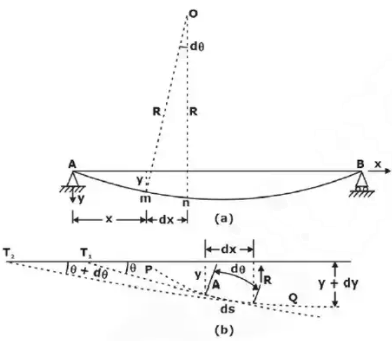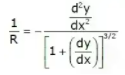Methods of Determining Beam Deflections

Numerous methods are available for the determination of beam deflections. These methods include:

1. Double Integration Method
• This is most suitable when concentrated or udl over entire length is acting on the beam. A double integration method is a powerful tool in solving deflection and slope of a beam at any point because we will be able to get the equation of the elastic curve.
• A double integration method is a powerful tool in solving deflection and slope of a beam at any point because we will be able to get the equation of the elastic curve.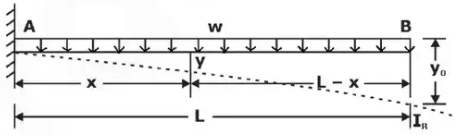• In calculus, the radius of curvature of a curve y = f(x) is given by: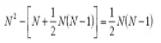• In the derivation of flexure formula, the radius of curvature of a beam is
ρ = EI / M

• Deflection of beams is so small, such that the slope of the elastic curve dy/dx is very small, and squaring this expression the value becomes practically negligible, hence: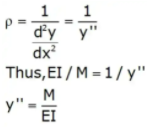• If EI is constant, the equation may be written as:
EIy′′ = M
where x and y are the coordinates shown in the figure of the elastic curve of the beam under load.

• y is the deflection of the beam at any distance x.

• E is the modulus of elasticity of the beam,

• I represent the moment of inertia about the neutral axis, and

• M represents the bending moment at a distance x from the end of the beam.

The product EI is called the flexural rigidity of the beam.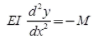Integrating one time: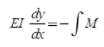The first integration y'(dy/dx) yields the Slope of the Elastic Curve.
Second Integration:

EI y = - ∫∫M

The second integration y gives the Deflection of the Beam at any distance x.

• The resulting solution must contain two constants of integration since EI y" = M is of second order.
• These two constants must be evaluated from known conditions concerning the slope deflection at certain points of the beam.
• For instance, in the case of a simply supported beam with rigid supports, at x = 0 and x = L, the deflection y = 0, and in locating the point of maximum deflection, we simply set the slope of the elastic curve y' to zero
2. Area Moment Method (Mohr's Method)
• Another method of determining the slopes and deflections in beams is the area-moment method, which involves the area of the moment diagram.The moment-area method is a
• The moment-area method is a semi graphical procedure that utilizes the properties of the area under the bending moment diagram. It is the quickest way to compute the deflection at a specific location if the bending moment diagram has a simple shape.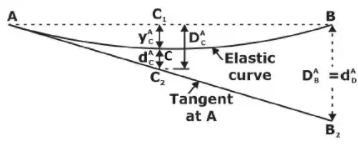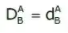(a) Theorems of Area-Moment Method

• Theorem 1: The angle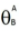between the tangent of the deflection curve of two points A and B is equal to the negative area of M/EI diagram between the points.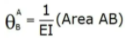• Theorem 2: The deviation of B from tangent at A is equal to the negative of the statical moment (or the first moment) with respect to B, of the M/EI diagram area between A and B.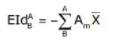3. Method of Superposition

The method of superposition, in which the applied loading is represented as a series of simple loads for which deflection formulas are available. Then the desired deflection is computed by adding the contributions of the component loads(principle of superposition).

• Mostly direct formula is used in questions, hence it is advised to look for the beam deflection formula which are directly asked from this topic rather than going for long derivations.

(i) Concentrated load at the free end of cantilever beam (origin at A)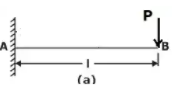• Maximum Moment: M = −PL
• Slope at end: θ = PL/ 2EI
• Maximum deflection: δ = PL/ 3EI
• Deflection Equation (y is positive downward): EIy = (Px2)(3L − x) / 6

(ii) Concentrated load at any point on the span of cantilever beam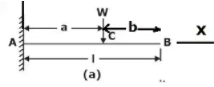• Maximum Moment: M = -wa
• Slope at end: θ = wa/ 2EI
• Maximum deflection: δ = wa3(3L − a) / 6EI
• Deflection Equation (y is positive downward):
(i) EIy = Px2(3a − x) / 6 for 0 < x <a
(ii) EIy = Pa2(3x − a) / 6 for a < x <L

(iii) Uniformly distributed load over the entire length of cantilever beam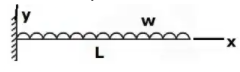• Maximum Moment: M = −wL/ 2
• Slope at end: θ = wL/ 6EI
• Maximum deflection: δ = wL/ 8EI
• Deflection Equation (y is positive downward): EIy = wx2(6L2− 4Lx + x2) / 120L

(iv) Triangular load, full at the fixed end and zero at the free end

•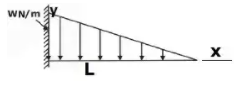Maximum Moment: M = −wL/ 6
• Slope at end: θ = wL/ 24EI
• Maximum deflection: δ = wL/ 30EI
• Deflection Equation (y is positive downward): EIy = wx2(10L3− 10L2x + 5Lx− x3) / 120L

(v) Moment load at the free end of cantilever beam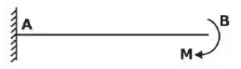• Maximum Moment: M = −M
• Slope at end: θ = ML / EI
• Maximum deflection: δ = ML/ 2EI
• Deflection Equation (y is positive downward): EIy = Mx/ 2

(vi) Concentrated load at the midspan of simple beam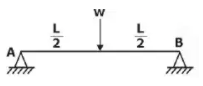• Maximum Moment: M = PL / 4
• Slope at end: θ= θB = WL2/ 16EI
• Maximum deflection: δ = PL3/ 48EI
• Deflection Equation (y is positive downward): EIy = Px{(3 / 4)L− x2)} / 12 for 0 < x < L / 2

(vii) Uniformly distributed load over the entire span of simple beam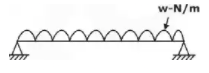• Maximum Moment: M = wL/ 8
• Slope at end: θL= θ= wL/ 24EI
• Maximum deflection: δ = 5wL/ 384EI
• Deflection Equation (y is positive downward): EIy = wx(L− 2Lx+ x3) / 24

(viii) Triangle load with zero at one support and full at the other support of simple beam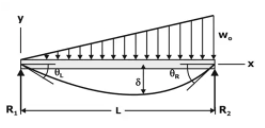• Maximum Moment: M = woL/ 9√3
• Slope at end:
(i) θL= 7wL/ 360EI
(ii) θ= 8wL/ 360EI
• Maximum deflection: δ = 2.5wL/ 384EI at x = 0.519L
• Deflection Equation (y is positive downward): EIy = wx(7L− 10L2x + 3x) / 360L

(ix) Triangular load with zero at each support and full at the midspan of simple beam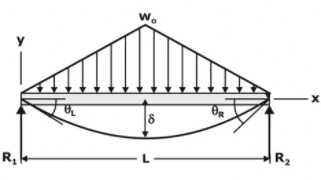• Maximum Moment: M = wL/ 12
• Slope at end: θL= θ= 5wL/ 192EI
• Maximum deflection: δ = wL/ 120EI
• Deflection Equation (y is positive downward): EIy = wox(25L− 40L2x+ 16x4) /960L    for 0 < x < L / 2

Conjugate Beam method (Method of elastic weights)

Rule.1: The slope at any point of a real beam, relative to the original axis of the beam, is equal to the shear force at the corresponding point of the conjugate beam.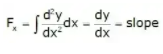Rule.2: The deflection at any point of a real beam, relative to the original axis of the beam is equal to the bending moment at the corresponding point of the conjugate beam.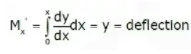Support conditions for the real and conjugate beam: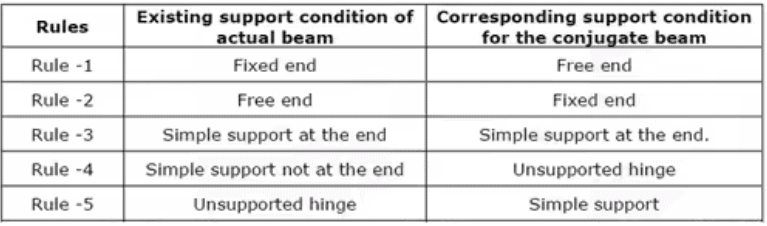Deflections by Castigliano's Theorem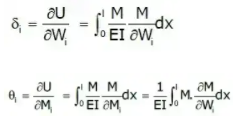Beam Deflection Formula
• Cantilever Beams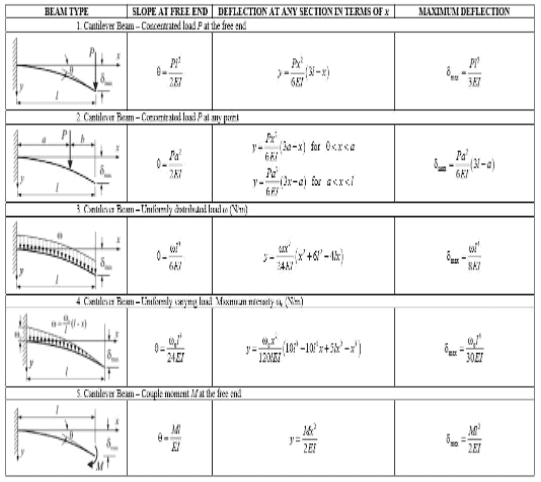• Simply supported Beams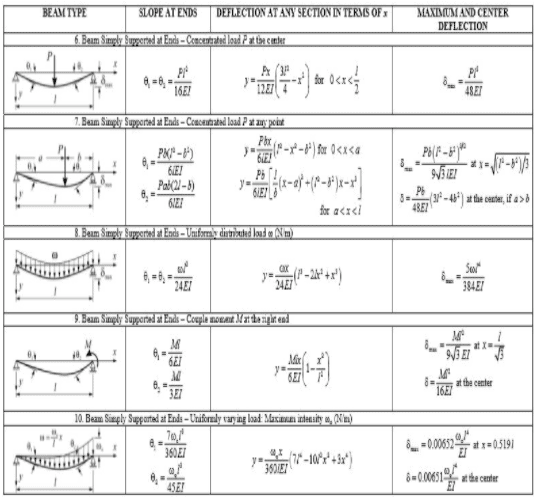Offer running on EduRev: Apply code STAYHOME200 to get INR 200 off on our premium plan EduRev Infinity!

## Strength of Materials (SOM)

35 videos|33 docs|48 tests

,

,

,

,

,

,

,

,

,

,

,

,

,

,

,

,

,

,

,

,

,

;